# Introduction

The rationale of graph4lg package in R is to make easier the construction and analysis of genetic and landscape graphs in landscape genetic studies (hence the name graph4lg, meaning Graphs for Landscape Genetics). This package provides users with tools for:

• Landscape and genetic data processing
• Genetic graph construction and analysis
• Landscape graph construction and analysis
• Landscape and genetic graph comparisons

Each one of the included tutorials focuses on one of these points. This fourth and last tutorial will then focus on landscape and genetic graph comparison. It will describe the package functions allowing users to:

• Compare node metrics
• Compare graph modules

## Data used in this tutorial

The package already includes genetic and spatial simulated data sets allowing users to discover its different functionalities. The first data set (data_simul) was simulated with CDPOP (Landguth and Cushman 2010) on a simulated landscape. It consists of 1500 individuals from 50 populations genotyped at 20 microsatellite loci. Individuals dispersed less when the cost-distance between populations was large. A landscape graph was created with Graphab (Foltête, Clauzel, and Vuidel 2012) whose nodes were the 50 simulated populations and the links were weighted by cost-distance values between populations. The project created with Graphab was included into the package such that the landscape graphs and the cost-distance matrix can be easily imported into the R environment.

The second data set (data_ex) was simulated as the first one but included only 10 populations. It is used to generate quick examples.

Here, we also rely on a data set created only for the vignettes (data_tuto) and containing several objects:

We will also use Graphab projects already created for the vignettes.

In landscape genetics, the analysis of the link between landscape and genetic data can be performed at several scales. Indeed, node-, link-, neighbourhood- and boundary-based analyses are distinguished (Wagner and Fortin 2013). Similarly, we can compare landscape and genetic graphs at these different scales, in particular when they share the same nodes. We present how to implement these comparisons using graph4lg. To see how to create landscape and genetic graphs with graph4lg, we invite users to read the second and third tutorials included in this package.

# Comparing node metrics

First, landscape and genetic graphs can be compared by comparing connectivity metrics measured at the level of a habitat patch (landscape graph node) with the genetic response of the population living and sampled in this habitat patch (genetic graph node) in terms of genetic diversity and differentiation from the other populations. When using this approach, two graphs are similar if the correlation coefficient between the metric values calculated for the same nodes is high. This correlation would be interpreted as an evidence for the influence of habitat connectivity on population genetic structure.

The function graph_node_compar computes these correlations to compare two graphs at the node level. It takes as arguments:

• x: A graph object of class igraph, i.e. the name of the first graph involved in the comparison. Its nodes must have the same names as in graph y.

• y: A graph object of class igraph, i.e. the name of the second graph involved in the comparison, sharing its nodes with graph x.

• metrics: A two-element character vector specifying the names of the node attributes from graphs x and y respectively, whose values will be used to assess their correlation. If these metrics are not within the node attributes, graph-theoretic metrics can be computed among:

• Degree ("deg"),
• Closeness centrality ("close"),
• Betweenness centrality ("btw"),
• Strength ("str"),
• Sum of inverse strength ("siw"),
• Mean of inverse link weight ("miw").
• method: A character string indicating which type of correlation coefficient is to be computed:

• Spearman’s rho (method="spearman",default)
• Pearson’r (method="pearson"),
• Kendall’s tau (method="kendall")
• weight: If new metrics are computed (see above), a logical (TRUE or FALSE) indicating whether the links are weighted during the calculation of the betweenness and closeness centrality indices. Link weights are interpreted as distances when computing the shortest paths.

• test: A logical (TRUE or FALSE) which indicates whether a significance test is performed

In order to include node attributes in the correlation, users can use compute them and associate them with graph nodes with add_nodes_attr function (described in tutorial 2).

In the following example, we will import a landscape graph made of the habitat patches occupied by the 50 populations used in a gene flow simulation with CDPOP (pts_pop_simul and data_simul_genind data set). A genetic graph will be created from the simulated genetic data set. We will then compare two metrics computed at the node-level in these graphs.

land_graph is the landscape graph made of the forest habitat patches occupied by the 50 populations. Its 1225 links are weighted by cost-distances between patches (mat_ld). It is a complete graph.

land_graph <- gen_graph_topo(mat_w = mat_ld,
mat_topo = mat_ld,
topo = "comp")

# Plot the histogram of its link weights
plot_w_hist(graph = land_graph)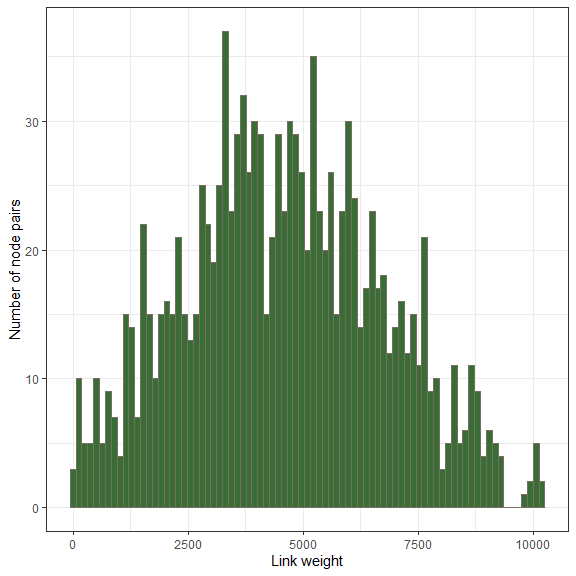Using the function plot_w_hist, we see that its link weights almost follow a normal distribution with values ranging from 0 to 10.000 cost-distance units.

We will compute the mean of the inverse weight of every link connected to its nodes. This metric miw is akin to the Flux metric computed in Graphab. It takes high values if a patch is well connected and close to other patches. We use the function compute_node_metric

miw_lg <- compute_node_metric(graph = land_graph, metrics = "miw")
head(miw_lg)

We include the values of this metric as a graph node attribute with the function add_nodes_attr:

land_graph <- add_nodes_attr(graph = land_graph,
input = "df",
data = miw_lg,
index = "ID")

We now create a genetic graph from the simulated data set. To that purpose, we first compute a genetic distance matrix using the DPS genetic distance.

mat_dps <- mat_gen_dist(x = data_simul_genind, dist = "DPS")

We use this distance matrix to create a complete genetic graph with gen_graph_topo:

gen_comp_graph <- gen_graph_topo(mat_w = mat_dps,
mat_topo = mat_dps,
topo = "comp")

We plot the distribution of the link weights.

plot_w_hist(graph = gen_comp_graph,
fill = "darkblue")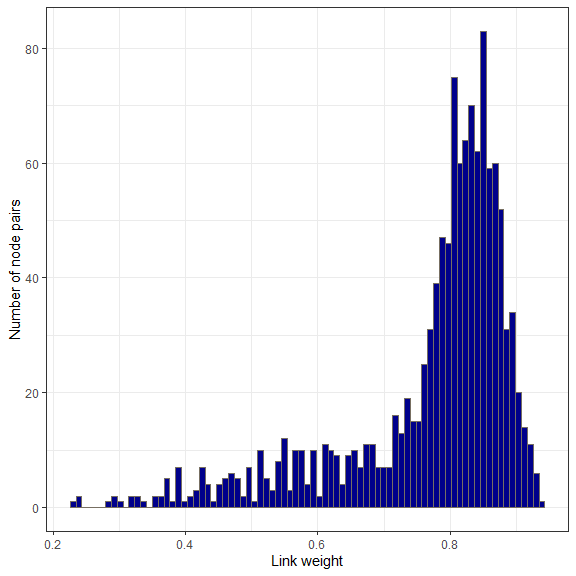We will compute the mean of the inverse weights of links connected to each node in the genetic graph gen_comp_graph and include it as a node attribute.

miw_comp <- compute_node_metric(graph = gen_comp_graph, metrics = "miw")
input = "df",
data = miw_comp,
index = "ID")

We can now assess the correlation between these two metrics using graph_node_compar:

graph_node_compar(x = land_graph, y = gen_comp_graph,
metrics = c("miw", "miw"), method = "spearman",
weight = TRUE, test = TRUE)
#> []
#>  "Metric from graph x: miw"
#>
#> []
#>  "Metric from graph y: miw"
#>
#> []
#>  "Method used: spearman's correlation coefficient"
#>
#> []
#>  "Sample size: 50"
#>
#> []
#>  "Correlation coefficient: 0.723793517406963"
#>
#> []
#>  "p-value of the significance test: 2.86499806974383e-09"

The two metrics are highly correlated, meaning that if a habitat patch is well connected, the population occupying this patch is less different from the other populations from a genetic point of view than if the habitat patch is not connected well. This is quite an expected result given that gene flow was largely driven by cost-distances between populations during the genetic simulations.

Using complete graphs, visual representations are unclear because of link overlap. Building a Gabriel graph is a way to simplify graph topology. Gabriel graphs links will be computed from the Euclidean geographical distances between populations.


mat_geo <- mat_geo_dist(data = pts_pop_simul,
ID = "ID", x = "x", y = "y")
#> Coordinates were treated as projected coordinates. Check whether
#>               it is the case.
mat_geo <- reorder_mat(mat_geo, order = row.names(mat_dps))

gen_gab_graph <- gen_graph_topo(mat_w = mat_dps,
mat_topo = mat_geo,
topo = "gabriel")

# Associate the values of miw from the complete graph to this graph

data = miw_comp,
index = "ID")

# Plot the graph with node sizes proportional to MIW

plot_graph_lg(graph = gen_gab_graph,
crds = pts_pop_simul,
mode = "spatial",
node_size = "miw",
link_width = "inv_w")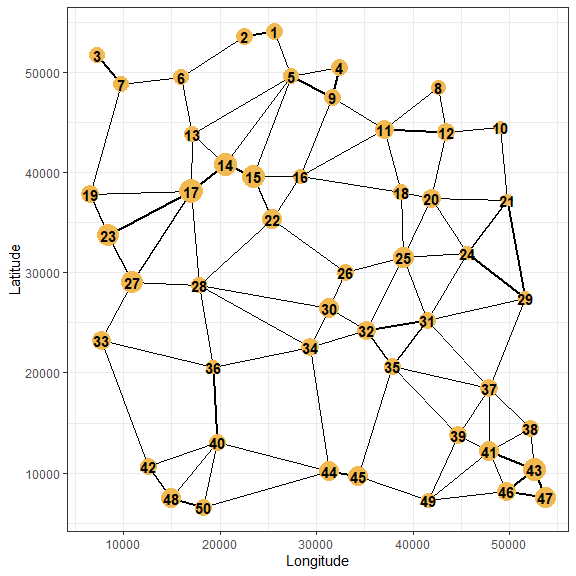# Comparing graph modules

Finally, two graphs have similar topological and connectivity properties if their modules match, i.e. if two nodes classified together in the same module when the partition in modules is computed from one graph are also classified together in the same module when the partition is computed from the other graph.

The function graph_modul_compar compares the nodes partitions into modules. To do that, it computes the Adjusted Rand Index (ARI) (Hubert and Arabie 1985), a standardised index which counts the number of node pairs classified in the same module in both graphs. The function also performs the nodes partition into modules by using the modularity calculations available in igraph package. We can specify the algorithm used to compute the modularity, the link weighting, the number of modules, among others. It takes as arguments:

• x: A graph object of class igraph, i.e. the name of the first graph involved in the comparison. Its nodes must have the same names as in graph y.

• y: A graph object of class igraph, i.e. the name of the second graph involved in the comparison, sharing its nodes with graph x.

• mode: The type of input data, which can be "graph" or data.frame or vector (see help file ?graph_modul_compar for more details).

• nb_modul: A numeric or integer value or a numeric vector with 2 elements indicating the number of modules to create in both graphs. By default, this number is the number of modules maximising modularity index.

• algo: A character string indicating the algorithm used to create the modules with igraph, among: "fast_greedy" (default), "walktrap", "louvain", "optimal".

• node_inter: A character string indicating whether the links of the graph are weighted by distances or by similarity indices. It is only used to compute the modularity index. It can be:

• 'distance': Link weights correspond to distances. Nodes that are close to each other will more likely be in the same module.
• 'similarity': Link weights correspond to similarity indices. Nodes that are similar to each other will more likely be in the same module. Inverse link weights are then used to compute the modularity index.
• 'none': Links are not weighted for the computation, which is only based on graph topology.

Two different weightings can be used to create the modules of the two graphs:

• If node_inter is a character string, then the same link weighting is used for both graphs.
• If node_inter is a character vector of length 2, then the link weighting used by the algorithm to create the modules of graphs x and y is determined by the first and second elements of node_inter, respectively.

In the following example, we compare land_graph_thr and gen_gab_graph with the default parameters (algo='fast_greedy' algorithm, optimal number of modules, links weighted by inverse distances (node_inter="distance")).

graph_modul_compar(x = land_graph_thr,
y = gen_gab_graph)
#>  "7 modules in graph 1 and 6 modules in graph 2"
#>  "Adjusted Rand Index: 0.648832401029973"
#>  0.6488324

The ARI value is relatively high meaning that modules are somehow similar.

We can check this result visually. We first compute the modules in both graphs and include them as node attributes.

module_land <- compute_graph_modul(graph = land_graph_thr,
algo = "fast_greedy",
node_inter = "distance")

data = module_land,
index = "ID")

module_gen <- compute_graph_modul(graph = gen_gab_graph,
algo = "fast_greedy",
node_inter = "distance")

data = module_gen,
index = "ID")

We then plot both graphs, colouring their nodes depending on their module:

plot_graph_lg(graph = land_graph_thr,
mode = "spatial",
crds = pts_pop_simul,
module = "module")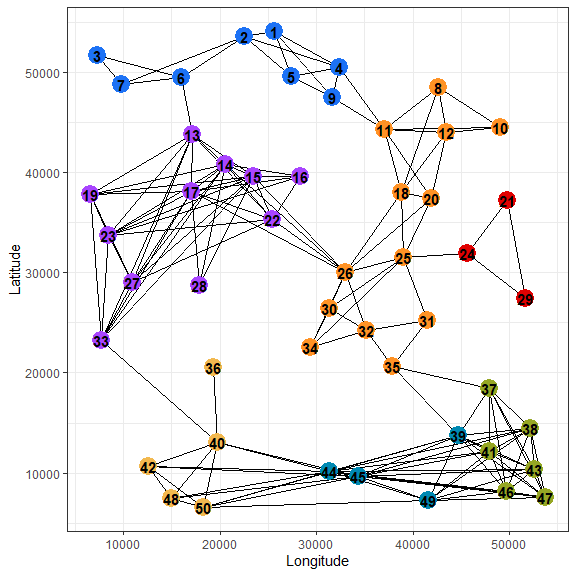plot_graph_lg(graph = gen_gab_graph,
mode = "spatial",
crds = pts_pop_simul,
module = "module")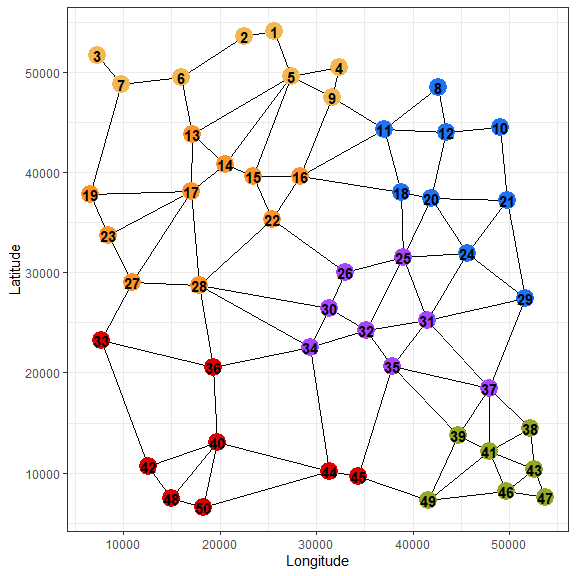We easily visualise divergence and convergence in both module partitions.

# Conclusion

graph4lg makes available a large range of tools to perform landscape genetic analyses relying upon genetic and landscape graphs. We hope that next improvements will draw on results obtained using these tools in empirical as well as simulation studies.